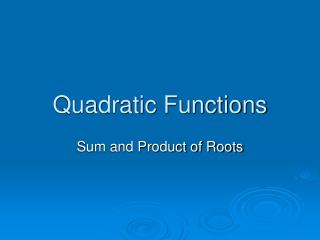DownloadDownload PresentationTélécharger la présentation- - - - - - - - - - - - - - - - - - - - - - - - - - - E N D - - - - - - - - - - - - - - - - - - - - - - - - - - -
##### Presentation Transcript

1. Quadratic Functions Sum and Product of Roots

2. Purpose • Find sum/product of roots without knowing the actual value of x. • Use the sum/product of roots to solve for other results

3. Derivation of Formula Given α and β are roots of a quadratic equation, we can infer that x1 = α and x2 = β , where α and β are just some numbers. For example, x2 – 5x + 6 = 0 has roots α and β means that α = 2 and β = 3

4. However… Sometimes, we do not need the values of x to help us solve the problem. Knowing the relationship between the quadratic equation and its roots helps us save time

5. Derivation of Formula ax2 + bx + c = 0 has roots α and β –(1) x2 + x + = 0 This tells us that: (x – α)(x – β) = 0 Expanding, we have: x2 – αx – βx + αβ = 0 x2– (α + β)x + αβ = 0 So, if we compare the above with (1): Result: αβ = , α + β =

6. In other words… ax2 + bx + c = 0 has roots α and β x2– (α + β)x + αβ = 0 x2– (sum of roots)x + product of roots = 0

7. Application – Example 1 (TB Pg 55) Hence, 2x2 + 6x – 3 = 0 has roots α and β, find the value of (a) Without finding α and β, we know the value for α + β and αβ α + β = – 3 and αβ = – 3/2

8. Application – Example 1 (TB Pg 55) 2x2 + 6x – 3 = 0 has roots α and β, find the value of (b) We already know that: α + β = – 3 and αβ = – 3/2 Expanding (b), we have

9. Application – Example 2 (TB Pg 55) x2 – 2x – 4 = 0 has roots α and β, find the value of (a) Step 1: α + β = 2 and αβ = – 4 To find (a), we use the algebraic property Rearranging:

10. Application – Example 2 (TB Pg 55) Hence, α − β = x2 – 2x – 4 = 0 has roots α and β, find the value of (b) Step 1: α + β = 2 and αβ = – 4 To find (b), we use the algebraic property Rearranging:

11. Application – Example 2 (TB Pg 55) x2 – 2x – 4 = 0 has roots α and β, find the value of (c) Step 1: α + β = 2 and αβ = – 4 To find (a), we use the algebraic property Rearranging:

12. Application – Example 3 (TB Pg 56) 2x2 + 4x – 1 = 0 has roots α and β, form the equation with roots α2 and β2 Remember! x2– (sum of roots)x + product of roots = 0 x2– (α2 + β2)x + α2β2 = 0 So in order to get the equation, I must find α2 + β2 and α2β2 How?